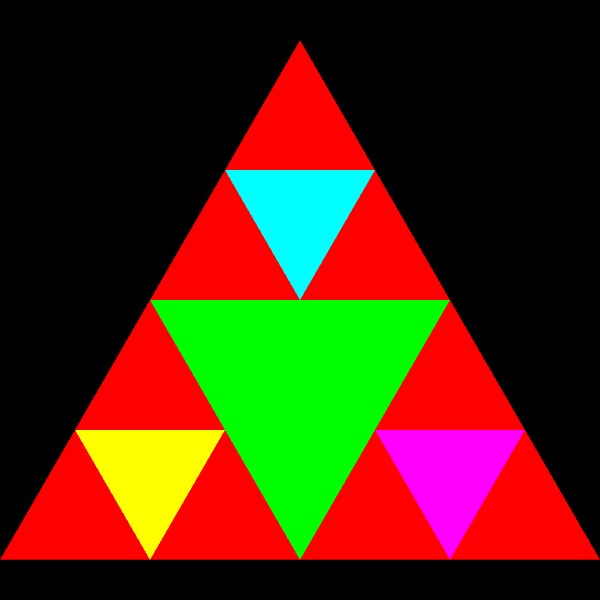UNB/ CS/ David Bremner/ teaching/ cs4735/ milestones/ CS4735 Assignment 2
• Available 2016-09-28
• Due 2016-10-07 17:30

### Before you start.

1. Make sure you can push to coursegit

2. See the assignment notes for the required repository structure.

3. Update your handouts repository; in the directory assignments/a2 you will find some template files for the last question on this assignment.

### Deliverables

For the first two "theoretical" parts, write your answers in Q{1,2}.{txt,odt,pdf}. You are welcome (and encouraged!) to use LaTeX, but please compile your TeX to PDF to save the TA trouble. You are encouraged, but not required, to provide sketches to help explain your answer.

For the third part, hand in a subdirectory Q3 containing any needed html and js files.

### Part 1. 2D transforms

Assume a 2D triangle with vertices at (1,0), (-1,0) and (0,1). Calculate the transformation matrices and the transformed coordinates (all in homogeneous coordinates) for the following transformations. Show your work.

1. Translate 1 unit to the left, rotate 90 degrees clockwise and translate 1 unit to the right.
2. Translate 1 unit to the right, rotate 90 degrees clockwise and translate 1 unit to the left.
3. Let `T1` and `T2` be the matrices from the first two parts. Find a transformation matrix `D` such that applying `D` after `T2` is the same as applying `T1`. Explain your answer in geometric terms.

### Part 2. 3D transforms

Let `S` be square in 3D with vertices `(0,0,1)`, `(0,1,1)`, `(1,0,1)` and `(1,1,1)`.

Give the transforms to map `S` to each face of the `0/1`-cube (this cube has all 8 3-bit numbers as coordinates). You may leave your transformations as products of primitive transformations.

### Part 3. Using 2D transforms

In the handouts repo, you will find a directory `assignments/a2` with most of the code to produce the figure below.

Copy the files to your assignment repo under directory `a2/Q3` and fill in the remaining calls to `drawTriangle`, along with appropriate transformation matrix changes to complete the figure below.